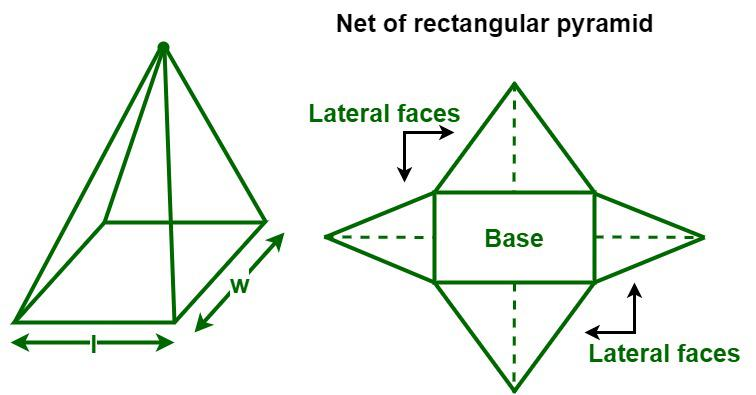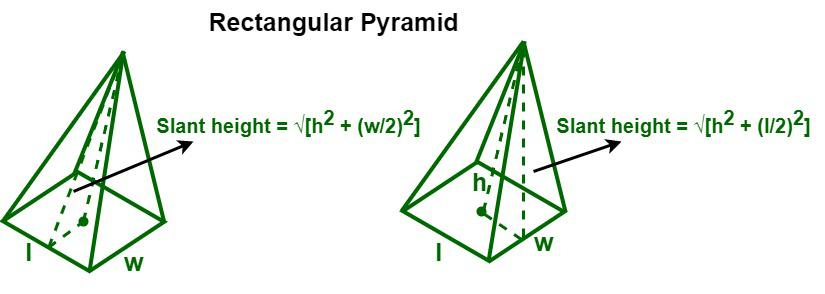Open in App
Not now

# Area of a Rectangular Pyramid

• Difficulty Level : Hard
• Last Updated : 01 Nov, 2022

A rectangular pyramid is a three-dimensional object that has a rectangular base upon which are erected four triangular faces that meet at a common point called the apex. It has a total of five faces, i.e., a rectangular base, four triangular faces, five vertices, and eight edges. In a rectangular pyramid, all the triangular faces are congruent to the opposite face. A rectangular pyramid is classified into two types, i.e., a right rectangular pyramid and an oblique rectangular pyramid. A right rectangular pyramid is a rectangular pyramid that has its apex directly above the center of its base, whereas an oblique rectangular pyramid is a rectangular pyramid where the apex is not aligned right above the center of its base. The height of an oblique rectangular pyramid is the perpendicular line drawn from the apex to the base of the pyramid. In this article, we will discuss the surface area of a rectangular pyramid in detail.## Surface Area of a Rectangular Pyramid

Surface area is defined as the total region occupied by the surfaces of a three-dimensional figure, and it is measured in terms of square units such as cm2, m2, in2, ft2, etc. A rectangular pyramid has two types of surface areas, i.e., the lateral surface area and the total surface area.

### Lateral Surface Area

The lateral surface area of a rectangular pyramid is equal to the sum of the areas of its four lateral faces (triangular faces). In a rectangular pyramid, the areas of the opposite triangular faces are the same. To find the surface area of a rectangular pyramid, we need to know the measures of the base length, base width, and slant height of the triangular face.

Lateral Surface Area of a Pyramid (LSA) = Sum of Areas of the lateral surfaces (triangles) of the pyramidSlant height of length face of the pyramid = √[h2 + (w/2)2]

Slant height of width face of the pyramid = √[h2 + (l/2)2]

We know that,

The area of a triangle = ½ × base × height

Area of the triangle that has a length as the base = ½ × l × {√[h2 + (l/2)2]}

Area of the triangle that has a width as the base = ½ × w × {√[h2 + (w/2)2}

Now, LSA = ½ × l × {√[h2 + (w/2)2]} + ½ × w × {√[h2 + (l/2)2} + ½ × l × {√[h2 + (w/2)2]} + ½ × w × {√[h2 + (l/2)2}

= 2 × {½ × l ×√[h2 + (w/2)2]} + 2 × {½ × w ×√[h2 + (l/2)2]}

= l√[h2 + (w/2)2] + w√[h2 + (l/2)2]

Lateral surface area of a rectangular pyramid = l√[h2 + (w/2)2] + w√[h2 + (l/2)2]

where,
“l” is the base length,
“w” is the base width, and
“h” is the height of the pyramid.

### Total Surface Area

The total surface area of a rectangular pyramid is equal to the sum of the areas of its four lateral faces (triangular faces) and the area of its rectangular base. To determine the total surface area of a rectangular pyramid, we need to find the area of its rectangular base and its lateral surface area, i.e., the sum of the areas of its four triangular faces.

Total surface area of a pyramid (TSA) = Lateral surface area of the pyramid + Area of the base

Area of rectangular base = l × w

So, TSA = l√[h2 + (w/2)2] + w√[h2 + (l/2)2] + l × w

TSA of a Rectangular Pyramid = l√[h2 + (w/2)2] + w√[h2 + (l/2)2] + l × w

where,

l” is the base length,
w” is the base width, and
h” is the height of the pyramid.

## How to Calculate the Surface Area of a Rectangular Pyramid?

The surface area of a rectangular pyramid is calculated by converting the 3-D shape into its 2-D net. After opening the Rectangular pyramid, we get four triangles and one rectangle. To find its surface area follow these steps.

Steps used to calculate the surface area of a rectangular pyramid is:

Step 1: Find the area of the rectangular base. The area of the rectangle is calculated using its length and breadth.

Step 2: Find an area of the triangle faces. The area of a triangle is calculated using its base and height.

Step 3: Now for finding the required surface area the area of the rectangular base and the triangular face are added accordingly.

Step 4: The area obtained in Step 3 is the required area it is measured in unit2

## Solved Examples on Surface Area of Rectangular Pyramid

Problem 1. Determine the lateral surface area of a rectangular pyramid if the base length is 10 inches and the base width is 8 inches, and the height of the pyramid is 12 inches.

Solution:

Given data,

Base length (l) = 16 inches

Base width (w) = 12 inches

The height of the pyramid (h) = 15 inches

We know that,

Lateral surface area of a rectangular pyramid = l√[h2 + (w/2)2] + w√[h2 + (l/2)2]

= 10 × √[122 + (8/2)2] + 8 × √[122 + (10/2)2]

= 10 × √(144 + 16) + 8 × √(144 + 25)

= 10 × √160 + 8 × √169

= 10 × 12.649 + 8 × 13

= 126.49 + 104 = 230.49 sq. in

Hence, the lateral surface area of the given rectangular pyramid is 230.49 sq. in.

Problem 2. Find the surface area of a rectangular pyramid if the base length is 8 cm and the base width is 6 cm, and the height of the pyramid is 10 cm.

Solution:

Given data,

Base length (l) = 8 cm

Base width (w) = 6 cm

The height of the pyramid (h) = 10 cm

We know that,

Total surface area of a rectangular pyramid = l√[h2 + (w/2)2] + w√[h2 + (l/2)2] + l × w

= (8 × √[102 + (6/2)2] + 6 × √[102 + (8/2)2] + 8 × 6

= 8 × √(100 + 9) + 6 × √(100 + 16) + 48

= 8 × √109 + 6 × √116 + 48

= 8 × 10.440 + 6 × 10.770 + 48

= 83.522 + 64.621 + 48 = 196.143 sq. cm

Hence, the surface area of the given rectangular pyramid is 196.143 sq. cm.

Problem 3. Find the total surface area of a rectangular pyramid if the base length is 12 cm and the base width is 10 cm, and the height of the pyramid is 15 cm.

Solution:

Given data,

Base length (l) = 12 cm

Base width (w) = 10 cm

The height of the pyramid (h) = 15 cm

We know that,

Total surface area of a rectangular pyramid = l√[h2 + (w/2)2] + w√[h2 + (l/2)2] + l × w

= (10 × √[152 + (12/2)2] + 12 × √[152 + (10/2)2] + 12 × 10

= 10 × √(225 + 36) + 12 × √(225 + 25) + 120

= 10 × √261 + 12 × √250 + 120

= 10 × 16.155 + 12 × 15.811 + 120

= 161.554 + 189.736 + 120 = 471.29 sq. cm

Hence, the surface area of the given rectangular pyramid is 471.29 sq. cm.

Problem 4. Determine the lateral surface area of a rectangular pyramid if the base length is 8 m and the base width is 4 m, and the height of the pyramid is 9 m.

Solution:

Given data,

Base length (l) = 8 m

Base width (w) = 4 m

The height of the pyramid (h) = 9 m

We know that,

Lateral surface area of a rectangular pyramid = l√[h2 + (w/2)2] + w√[h2 + (l/2)2]

= 8 × √[92 + (4/2)2] + 4 × √[92 + (8/2)2]

= 8 × √(81 + 4) + 4 × √(81 + 16)

= 8 × √85 + 4 × √97

= 8 × 9.219 + 4 × 9.849

= 73.756 + 39.395 = 113.151 sq. m

Hence, the lateral surface area of the given rectangular pyramid is 113.151 sq. m.

Problem 5. Find the surface area of a rectangular pyramid if the base length is 20 inches and the base width is 16 inches, and the height of the pyramid is 25 inches.

Solution:

Given data,

Base length (l) = 20 inches

Base width (w) = 16 inches

The height of the pyramid (h) = 25 inches

We know that,

Total surface area of a rectangular pyramid = l√[h2 + (w/2)2] + w√[h2 + (l/2)2] + l × w

= (20 × √[252 + (16/2)2] + 16 × √[252 + (20/2)2] + 20 × 16

= 20 × √(625 + 36) + 16 × √(625 + 100) + 320

= 20 × √689 + 16 × √250 + 320

= 20 × 26.249 + 16 × 26.925 + 320

= 524.976 + 430.813 + 320 = 1,275.789 sq. in

Hence, the surface area of the given rectangular pyramid is 1,275.789 sq. in.

## FAQs on Surface Area of Rectangular Pyramid

Question 1: What is the definition of a rectangular pyramid?

A rectangular pyramid is a three-dimensional geometric figure that has a rectangular base and four triangular faces that meet at a common vertex called the apex.

Question 2: What are the types of rectangular pyramids?

There are two types of rectangular pyramids, namely, a right rectangular pyramid and an oblique rectangular pyramid.

Question 3: What is the formula to find the lateral surface area of a rectangular pyramid?

The lateral surface area of a rectangular pyramid is equal to the sum of the areas of its four lateral faces (triangular faces), and the formula to calculate it is given below.

Lateral surface area of a rectangular pyramid = l√[h2 + (w/2)2] + w√[h2 + (l/2)2]

Where “l” is the base length,

“w” is the base width, and

“h” is the height of the pyramid.

Question 4: Define surface area.

Surface area is defined as the total region occupied by the surfaces of a three-dimensional figure.

Question 5: What is the formula to find the total surface area of a rectangular pyramid?

The total surface area of a rectangular pyramid is equal to the sum of the areas of its four lateral faces (triangular faces) and the area of its rectangular base, and the formula to calculate it is given below.

Total surface area of a rectangular pyramid = l√[h2 + (w/2)2] + w√[h2 + (l/2)2] + l × w

where,
l” is the base length,
w” is the base width,
h” is the height of the pyramid.

### Related Resources

My Personal Notes arrow_drop_up